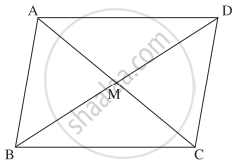# Prove that a quadrilateral is a parallelogram if and only if its diagonals bisect each other - Mathematics and Statistics

Sum

Prove that a quadrilateral is a parallelogram if and only if its diagonals bisect each other.

#### Solution

(i) Let bar"a", bar"b", bar"c"  "and"  bar"d" be respectively the position vectors of the vertices A, B, C and D of the parallelogram ABCD.
Then AB = DC and side AB || side DC.∴ bar"AB" = bar"DC"

∴ bar"b" - bar"a" = bar"c" - bar"d"

∴ bar"a" + bar"c" = bar"b"  bar"d"

∴ (bar"a" + bar"c")/2 = (bar"b" + bar"d")/2   ....(1)

The position vectors of the midpoints of the diagonals AC and BD are (bar"a" + bar"c")/2  and  (bar"b" + bar"d")/2.

By (1), they are equal.

∴ the midpoints of the diagonals AC and BD are the same.

This shows that the diagonals AC and BD bisect each other.

(ii) Conversely, suppose that the diagonals AC and BD of square ABCD bisect each other,
i.e. they have the same midpoint.

∴ the position vectors of these midpoints are equal.

∴ (bar"a" + bar"c")/2 = (bar"b" + bar"d")/2

∴ bar"a" + bar"c" = bar"b" + bar"d"

∴ bar"b" - bar"a" = bar"c" - bar"d"

∴ bar"AB" = bar"DC"

∴ bar"AB" || bar"DC" and |bar"AB"| = |bar"DC"|

∴ side AB || side DC and AB = DC

∴ square "ABCD" is a parallelogram.

Concept: Section Formula
Is there an error in this question or solution?